# How Geodesic Viewshed works

The Geodesic Viewshed tool determines surface locations visible to a set of point or polyline observers, using geodesic methods. It transforms the elevation surface into a geocentric 3D coordinate system and runs 3D sightlines to each transformed cell center. It will take advantage of a graphics processing unit (GPU), if available on your system. It optionally accommodates vertical uncertainty or error in the input elevation surface. It also optionally produces an observer-region relationship table for up to 32 observers (points, multipoints, or polylines) that can be related back to the input observer feature class.

Since the computation is performed in a true 3D coordinate system, Geodesic Viewshed does not need the earth curvature correction parameter. It also uses the z units of the input spatial reference, if available, rather than a z-factor parameter. Finally, since each 3D sightline is evaluated independently of other sightlines, it avoids some of the errors that can creep into a wavefront-based viewshed algorithm (such as the existing viewshed family of geoprocessing tools). Thus, the Geodesic Viewshed tool produces more accurate visibility and AGL surfaces than the Viewshed tool.

## The determination of visibility

This tool uses geocentric 3D points for observers, targets, and elevation surface cell centers. A target is a surface cell center that has an optional, additional offset added to it. A geocentric 3D coordinate system is a right-handed Cartesian coordinate system with the center of the earth as the origin C, the x axis pointing to the intersection of the prime meridian and the equator, the Z axis pointing to the North Pole, and the y axis rotated 90° clockwise from the x axis while looking down from the North Pole. See the following figure for an example of a target location T expressed with geocentric coordinates.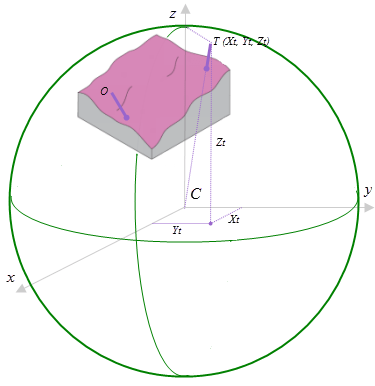The surface raster and the observers are transformed from the input coordinate system into a 3D geocentric coordinate system. Target T’s geocentric coordinates are (Xy, Yt, Zt).

To determine the visibility of each target, 3D sightlines from each observer to each target are constructed. See the figure below for an illustration of how the sightlines are constructed. The ground path of each sightline on the spheroid is determined and divided into cell size steps. In this figure, the steps are shown as the green dots, and the distance between them is equivalent to the cell size. At each step, the distance d along the normal to the spheroid, between the sightline and the surface, is calculated. The elevation of ground is estimated using a linear Inverse Distance Weighted (IDW) approach using the nearest cell centers. If d is positive for all ground steps along the sightline, then the target is considered visible; otherwise, it is considered not visible.

The ground path of each sightline on the spheroid is determined and divided into cell size steps. At each step, the distance d along the normal to the spheroid, between the sightline and the surface, is calculated. The elevation of ground is estimated using a linear IDW approach using the nearest cell centers. If d is positive for all ground steps along the sightline, then the target is considered visible; otherwise, it is considered not visible.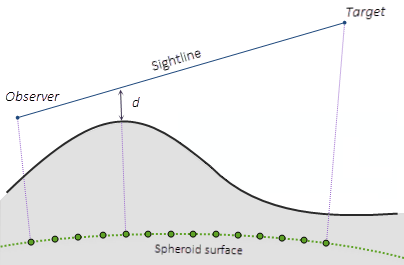Profile view of a geocentric 3D sightline, shown relative to the elevation surface and the underlying spheroid

## Vertical error

The Vertical error parameter is only enabled when the analysis type is Frequency. It is used to account for the vertical uncertainty in the input elevation surface. When this parameter is 0 or not specified, a single sightline is cast between the observer and each target. The result is that the target is either visible or not visible. In this case, the output visibility raster records the number of times that each cell location in the input surface raster can be seen by any of the input observation points.

When the Vertical error parameter is greater than 0 (for example, 0.6 meters), some number of sightlines are cast between the observer and each target. For each sightline and each step, a uniformly distributed random number in the range of [-0.6, 0.6] is added to d. If d becomes less than zero, that particular sightline is terminated. In this case, each observer contributes a number between zero and one (the number of successful sightlines divided by the total number of sightlines, from that observer) to the output visibility raster, which is now of type single-precision float.

## The determination of AGL

An Above Ground Level (AGL) raster is an optional output that represents the above ground height that the nonvisible target cells would need to be raised by in order to become visible. The following figure illustrates how the AGL value is determined. T is a target with a certain height (which is the target offset in the illustration). The sightline between the observer O and the target T is blocked by the terrain, so target T is not visible. If the target is raised to the new position T’, then it becomes visible because there is now a clear sightline. The distance between T’ and the ground is the AGL value in the output raster.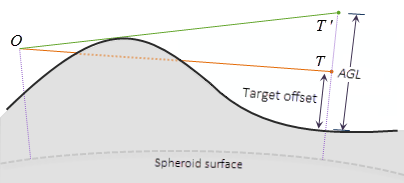The AGL output raster records the additional elevation above the surface needed to make invisible targets visible to at least one observer. In this example, the AGL value at the target location is target offset + TT'.

The computation of distance TT' does not assume that triangle OTT' is a right triangle.

## Interpreting the output observer-region relationship table

When the analysis type is Observers, the tool outputs an observer-region relationship table, as shown in the following figure. In this table, the Observer field lists feature IDs from the input feature class. The Region field lists cell values for regions in the output visibility raster. Thus, the table lists the regions that are visible to each observer. You can use this table to look up the region IDs given an observer ID, and vice versa.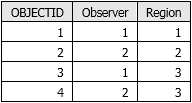The observer-region relationship table identifies which features can see which part of the surface.

The same information is encoded in the output cell values of the visibility raster, but in a less accessible way. In this case, the output visibility raster contains the three values (1, 2, and 3), as shown in the following screen capture of the attribute table.

The input observers are sorted in ascending order by their OBJECTID values, and the output visibility raster reports the bitwise OR of their sorted positions. Raster Map Algebra can be used to extract information about which observers can see which regions, and that information can be related back to observer features using this sorting rule. An example is shown in the figure below. Assume that Observer 1 has ObjectID 1 and Observer 2 has ObjectID 2. Then Region 1 (the pale yellow area) in the output visibility map will have a cell value of 1. Region 2 (the blue area) in the output visibility map will have a cell value of 2. And Region 3 (the green area) in the output visibility map will have a cell value of 3, the numeric value of the bitwise OR of 1 and 2.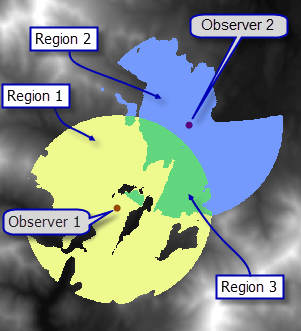An example of the output for two observers, when the Observer analysis type is selected instead of Frequency

In the illustration above, the output cell values are the bitwise OR of the internal indexes of the input features. The observer-region relationship table reports this same information using feature class IDs instead of internal indexes.

## Controlling the visibility analysis

It is possible to limit the field of view for each observer by specifying various numerical values or fields in the observer parameters. These observer parameters are functionally the same as the view cone fields—such as OFFSETA or OFFSETB—used for controlling the visibility analysis by the Viewshed tool. The difference is that the Geodesic Viewshed observer parameters can take any numerical fields and are not limited to any specific field names.

## Use of a GPU

This tool is capable of delivering increased performance if you have certain GPU hardware installed on your system. See the GPU Processing with Spatial Analyst topic for more details on how this is supported, how to configure it, and how to enable it.